# th 8 Grade Equations and Inequalities Review RULES

• Slides: 23
Download presentation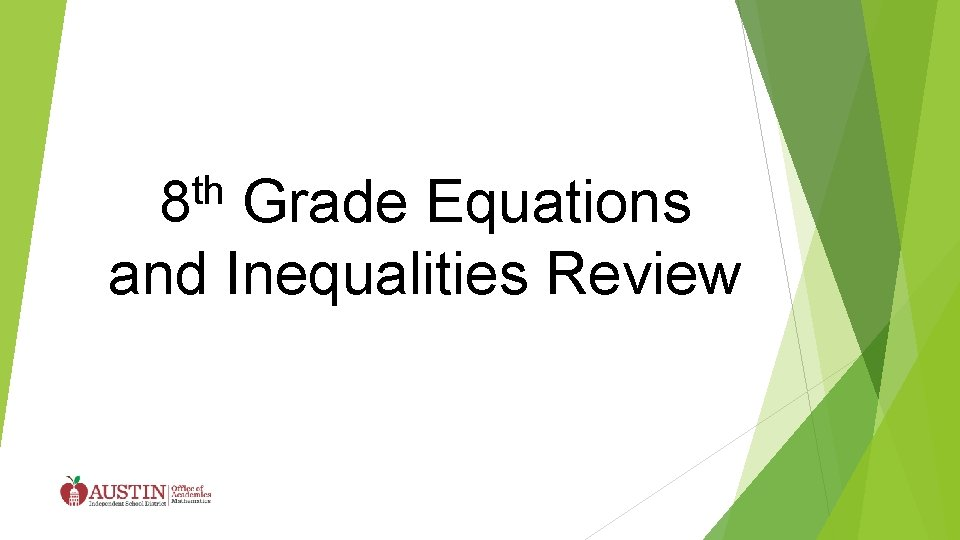th 8 Grade Equations and Inequalities ReviewRULES Start with \$1000 Use your money wisely!!!! You can only bet up to \$100 less than your total. Make a bet on each problem. If you get the question correct add it to your total. If you get the question wrong you lose your bet and must subtract it from your total. BE HONEST!!!!!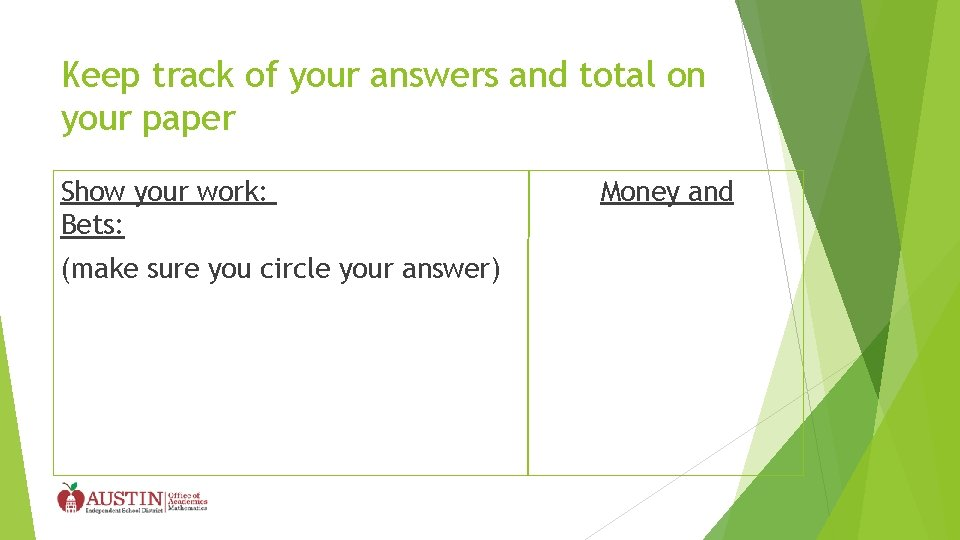Keep track of your answers and total on your paper Show your work: Bets: (make sure you circle your answer) Money andQuestion 1 The following scale is balanced. Find the value x, of the green rectangle.Answer x = -2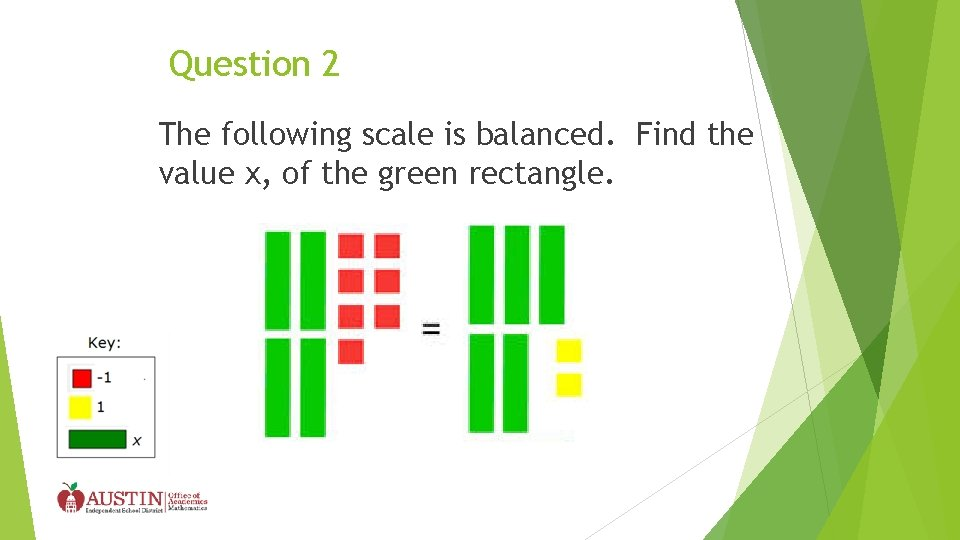Question 2 The following scale is balanced. Find the value x, of the green rectangle.Answer x = -9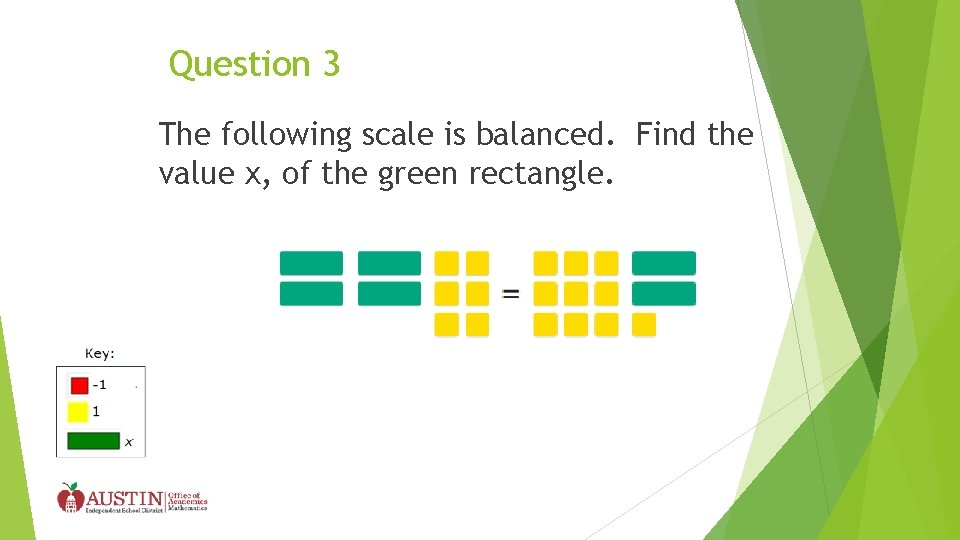Question 3 The following scale is balanced. Find the value x, of the green rectangle.Answer x=2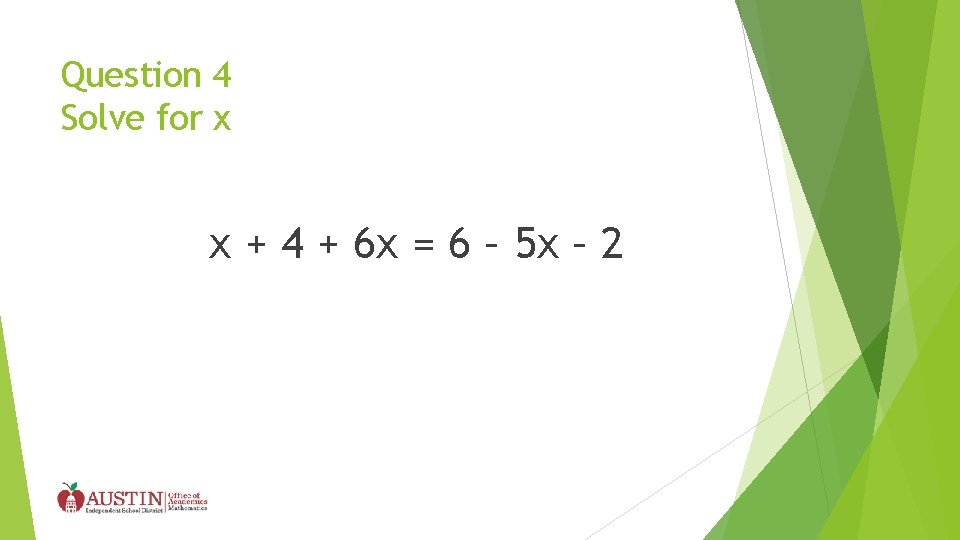Question 4 Solve for x x + 4 + 6 x = 6 – 5 x – 2Answer x=0Question 5 Solve for x 7 x + 5 = 6 x -3 AISD MIddle School Math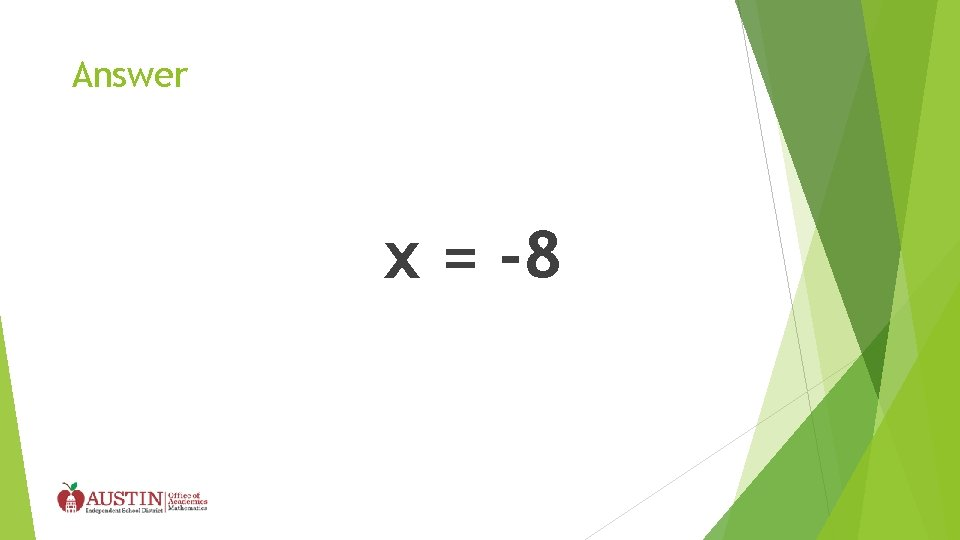Answer x = -8Question 6 Solve the equation for the given variable. 6 j + 4 = 2( j – 4 )Answer j = -3Question 7 Ana is saving for a summer vacation that cost no less than \$350. She has \$100 saved and hopes to babysit for \$8 per hour to earn the rest of the money. Write an inequality to describe the number of hours, h, she must babysit to have enough money for the trip.Answer 100 + 8 h > 350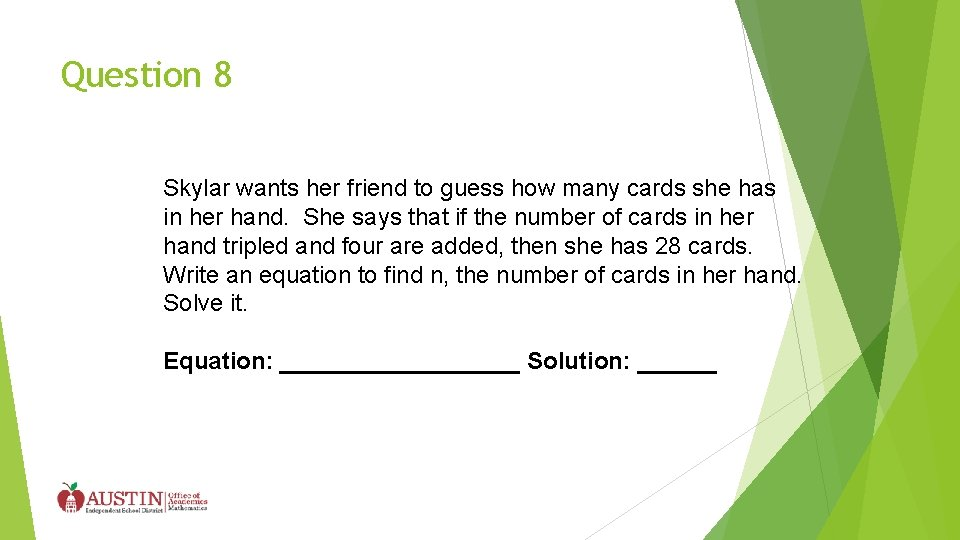Question 8 Skylar wants her friend to guess how many cards she has in her hand. She says that if the number of cards in her hand tripled and four are added, then she has 28 cards. Write an equation to find n, the number of cards in her hand. Solve it. Equation: _________ Solution: ______Question 8 Answer Equation: 3 x + 4 = 28 Solution: x = 8 cardsQuestion 9 Monica works after school and on weekends at the mall. She earns \$7. 50 per hour. Last week, she earned at least \$95. Write an inequality that can be used to find h, the number of hours she worked last week.Answer 7. 50 h > 95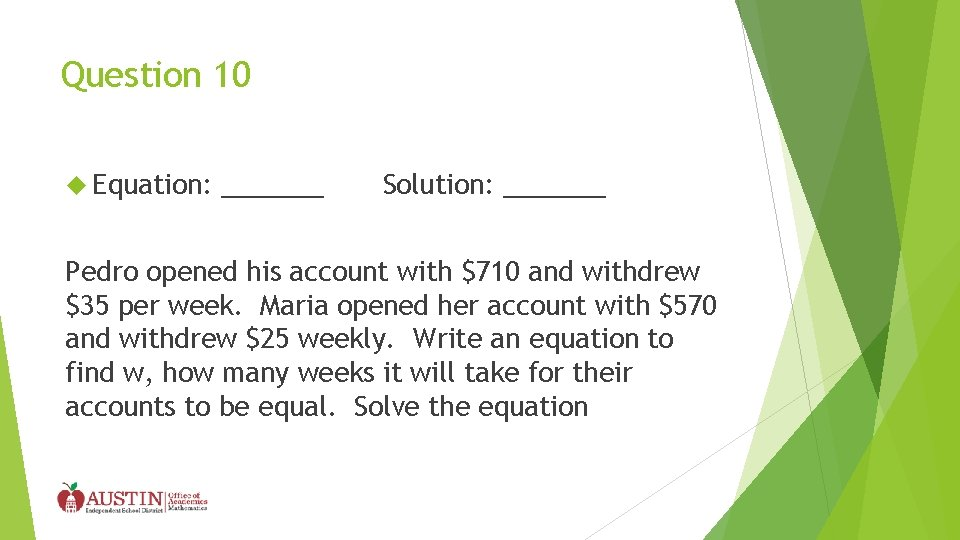Question 10 Equation: _______ Solution: _______ Pedro opened his account with \$710 and withdrew \$35 per week. Maria opened her account with \$570 and withdrew \$25 weekly. Write an equation to find w, how many weeks it will take for their accounts to be equal. Solve the equationAnswer Equation: Solution: 710 – 35 w = 570 – 25 w 14 Weeks